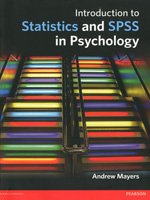Introduction to Statistics & SPSS in Psychology

By Andrew Mayers
August 2013
Pearson Education
Distributed by Trans-Atlantic Publications
ISBN 9780273731016
611 Pages, Illustrated
\$87.50 Paper originalIntroduction to Statistics and SPSS in Psychology guides the reader carefully and concisely up the statistics staircase to success. Each step is supported by helpful visuals as well as advice on how to overcome problems. Interactive, lively, but never patronising, this is the complete guide to statistics that will take readers through their degree course from beginning to end.

Take a step in the right direction and tackle statistics head on with this visual introduction.

Contents:

1. Introduction

2. SPSS: The Basics

3. Normal Distribution

4. Significance, effect size, and power

5. Experimental methods - how to choose the correct statistical test

6. Correlation

7. Independent t-test

8. Related t-test

9. Independent one-way ANOVA

10. Repeated-measures one-way ANOVA

11. Independent multi-factorial ANOVA

12. Repeated-measures multi-factorial ANOVA

13. Mixed multi-factorial ANOVA

14. Multivariate analyses

15. Analyses of covariance

16. Linear and multiple linear regression

17. Logistic regression

18. Non-parametric tests

19. Tests for categorical variables

20. Factor analysis

21. Reliability analysis